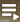# Ukuqonda Video Presentations

Included are series of video lesson covering high leverage Mathematics content areas that serve as:

• threshold concepts (doors to other content) for learners, and
• vehicles for teacher change towards more ambitious teaching (providing space for and taking note of learner constructions, aiming for understanding of concepts).

This is and will remain a developing space where new video lessons will be posted and made available for both educators and learners. The ideas articulated are mostly based on the  Ukuqonda material as written up in our Ukuqonda/Sasol-Inzalo grades 4 to 6 and grades 7 to 9 Mathematics textbooks and teacher guides.

### Series on the Introduction to Fractions

The Introductory 10 lessons on fractions can be viewed from the following YouTube playlist.

The video will start with lesson 1 and play on to 10....

Selecting a specific lesson - In the top right-hand corner of the video screen select the following icon to choose any of the 10 lessons:Have fun!

The concepts of what a fraction is, and what equivalent fractions are, form the core of this series. It is aimed at anyone wanting to improve their understanding of fractions. In the South African curriculum(CAPS) it would be suitable for learners from Grade 4 and onward. The series is made up of the followiing 10 lessons:

1. Looking at how we use numbers or quantities to describe a number of objects as this is an important principle to understand when describing fractions.
2. Explaining what a fraction is and introduces the language of fractions, in particular in naming unit (one part of a fraction) fractions.
3. Revisits the describe of a number of objects from lesson 1 and applies this to describing more than one part of a fraction.
4. Starts to discuss equivalent fractions in looking at different ways of describing the same fraction. This lesson is split into two parts. Part A looks at equivalent fractions of common fractions and wholes and Part B looks at equivalent mixed numbers and improper fractions (without introducing that terminology yet).
5. Discusses finding the fraction of a number bigger than 1. There are a few questions at the end that can be completed for discussion in the next lesson.
6. Goes over the questions from lesson 5 with complete solutions and a discussion on each question.
7. Introduces the use of fraction symbols. Up until now we have only been using fraction language to name and describe fractions. In this lesson content from the first 3 lessons is re-visited while implementing fraction symbols.
8. Revisits the concept of equivalent fractions from lesson 4 on mixed numbers and improper fractions and introduces the terminology of these two equivalent forms and moving between the forms.
9. Expands on what was introduced in lesson 8 and takes the equivalent fractions of improper fractions and mixed numbers further. There are questions at the end to complete for discussion in the next lesson.
10. The final lesson in this series works through solutions and thinking around the questions given at the end of lesson 9.